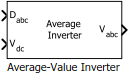Average-Value Inverter

Compute three-phase AC voltage from inverter DC voltage

• Library:
• Motor Control Blockset / Electrical Systems / Inverters

•Description

The Average-Value Inverter block models an average-value and full-wave inverter. It computes the three-phase AC voltage output from inverter DC voltage by using the duty cycle information.

Equations

These equations describe how the block computes the three-phase AC voltage.

${D}_{0}=\frac{\left({D}_{a}+{D}_{b}+{D}_{c}\right)}{3}$

${V}_{a}={V}_{dc}×\left({D}_{a}-{D}_{0}\right)$

${V}_{b}={V}_{dc}×\left({D}_{b}-{D}_{0}\right)$

${V}_{c}={V}_{dc}×\left({D}_{c}-{D}_{0}\right)$

where:

• ${D}_{a}$, ${D}_{b}$, and ${D}_{c}$ are the modulation indices ranging between 0 and 1.

• ${V}_{dc}$ is the DC bus voltage of the inverter (Volts).

• ${V}_{a}$, ${V}_{b}$, and ${V}_{c}$ are the output three-phase voltages (Volts).

Ports

Input

expand all

Three-phase modulation indices in the range [0,1] for generating voltages that run the motor.

Data Types: single | double | fixed point

DC bus voltage input to the inverter.

Data Types: single | double | fixed point | uint8 | uint16 | uint32

Output

expand all

Three-phase voltage (Volts) corresponding to the input duty cycle that runs the motor.

Data Types: single | double | fixed point